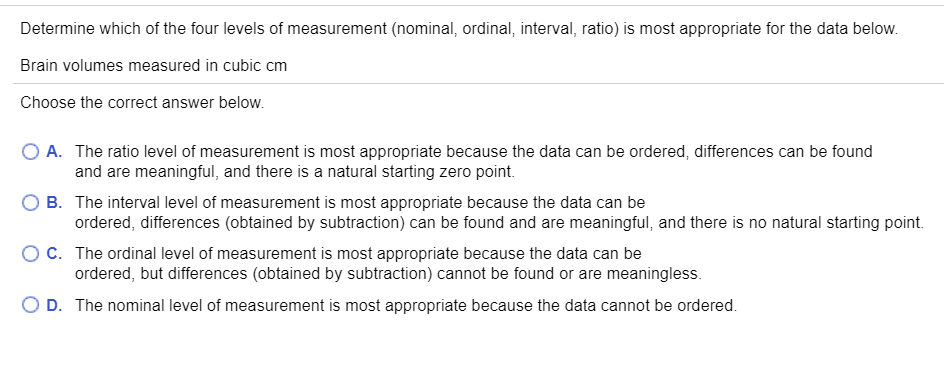# Determine which of the four levels of measurement (nominal, ordinal, interval, ratio) is most appropriate for the data below. Brain volumes measured in cubic cm Choose the correct answer below. A. The ratio level of measurement is most appropriate because the data can be ordered, differences can be found and are meaningful, and there is a natural starting zero point. O B. The interval level of measurement is most appropriate because the data can be ordered, differences (obtained by subtraction) can be found and are meaningful, and there is no natural starting point. C. The ordinal level of measurement is most appropriate because the data can be ordered, but differences (obtained by subtraction) cannot be found or are meaningless. O D. The nominal level of measurement is most appropriate because the data cannot be ordered.

Questionhelp_outlineImage TranscriptioncloseDetermine which of the four levels of measurement (nominal, ordinal, interval, ratio) is most appropriate for the data below. Brain volumes measured in cubic cm Choose the correct answer below. A. The ratio level of measurement is most appropriate because the data can be ordered, differences can be found and are meaningful, and there is a natural starting zero point. O B. The interval level of measurement is most appropriate because the data can be ordered, differences (obtained by subtraction) can be found and are meaningful, and there is no natural starting point. C. The ordinal level of measurement is most appropriate because the data can be ordered, but differences (obtained by subtraction) cannot be found or are meaningless. O D. The nominal level of measurement is most appropriate because the data cannot be ordered. fullscreen

### Want to see this answer and more?

Experts are waiting 24/7 to provide step-by-step solutions in as fast as 30 minutes!*

*Response times may vary by subject and question complexity. Median response time is 34 minutes for paid subscribers and may be longer for promotional offers.
Tagged in
Math
Statistics

### Other